JEE  >  JEE Main Previous year questions (2021-22): Properties of Matter - 1

# JEE Main Previous year questions (2021-22): Properties of Matter - 1 - Notes | Study Physics 35 Years JEE Main & Advanced Past year Papers - JEE

 1 Crore+ students have signed up on EduRev. Have you?

Q.1. A bubble has surface tension S. The ideal gas inside the bubble has ratio of specific heats γ = 5/3. The bubble is exposed to the atmosphere and it always retains its spherical shape. When the atmospheric pressure is Pa1, the radius of the bubble is found to be r1 and the temperature of the enclosed gas is T1. When the atmospheric pressure is Pa2, the radius of the bubble and the temperature of the enclosed gas are r2 and T2, respectively.

Which of the following statement(s) is(are) correct?    (JEE Advanced 2022)
(a) If the surface of the bubble is a perfect heat insulator, then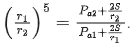(b) If the surface of the bubble is a perfect heat insulator, then the total internal energy of the bubble including its surface energy does not change with the external atmospheric pressure.
(c) If the surface of the bubble is a perfect heat conductor and the change in atmospheric temperature is negligible, then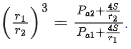(d) If the surface of the bubble is a perfect heat insulator, then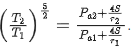Ans. c, d

Q.2. An ideal gas of density ρ = 0.2 kg m−3 enters a chimney of height h at the rate of α = 0.8 kg s−1 from its lower end, and escapes through the upper end as shown in the figure. The cross-sectional area of the lower end is A= 0.1 m2 and the upper end is A2 = 0.4 m2. The pressure and the temperature of the gas at the lower end are 600 Pa and 300 K, respectively, while its temperature at the upper end is 150 K. The chimney is heat insulated so that the gas undergoes adiabatic expansion. Take g = 10 m s−2 and the ratio of specific heats of the gas γ = 2. Ignore atmospheric pressure.    (JEE Advanced 2022)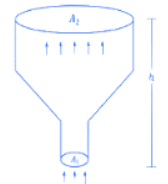Which of the following statement(s) is(are) correct?
(a) The pressure of the gas at the upper end of the chimney is 300 Pa.
(b) The velocity of the gas at the lower end of the chimney is 40 m s−1 and at the upper end is 20 ms−1.
(c) The height of the chimney is 590 m.
(d) The density of the gas at the upper end is 0.05 kg m−3.

Ans. b

Q.3. The speed of a transverse wave passing through a string of length 50 cm and mass 10 g is 60 ms−1. The area of cross-section of the wire is 2.0 mm2 and its Young's modulus is 1.2 × 1011Nm−2. The extension of the wire over its natural length due to its tension will be x × 10−5 m. The value of x is __________.    (JEE Main 2022)

Ans. 15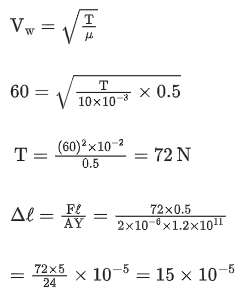Q.3. The velocity of a small ball of mass 0.3 g and density 8 g/cc when dropped in a container filled with glycerine becomes constant after some time. If the density of glycerine is 1.3 g/cc, then the value of viscous force acting on the ball will be x × 10−4 N, The value of x is _________. [use g = 10 m/s2]    (JEE Main 2022)

Ans. 25
F+ FB = mg(v= constant)
FV = mg − FB
= ρBVg − ρLVg
= (ρB−ρL)Vg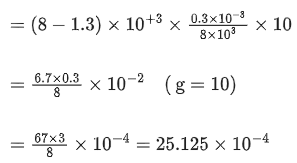Q.4. A metal wire of length 0.5 m and cross-sectional area 10−4 m2 has breaking stress 5 × 108Nm−2. A block of 10 kg is attached at one end of the string and is rotating in a horizontal circle. The maximum linear velocity of block will be _________ ms−1.    (JEE Main 2022)

Ans. 50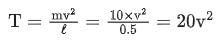Tmax =  Breaking stress × Area
= 5 × 108 × 10−4 = 5 × 104
20 V2 = 5 x 104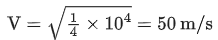Q.5. A tube of length 50 cm is filled completely with an incompressible liquid of mass 250 g and closed at both ends. The tube is then rotated in horizontal plane about one of its ends with a uniform angular velocity x√Frads−1. If F be the force exerted by the liquid at the other end then the value of x will be __________.    (JEE Main 2022)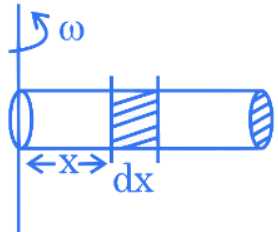Ans. 4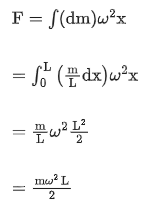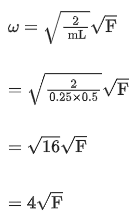Q.6. A string of area of cross-section 4 mm2 and length 0.5 m is connected with a rigid body of mass 2 kg. The body is rotated in a vertical circular path of radius 0.5 m. The body acquires a speed of 5 m/s at the bottom of the circular path. Strain produced in the string when the body is at the bottom of the circle is _________ ×10−5.
(use Young's modulus 1011 N/m2 and g = 10 m/s2)    (JEE Main 2022)

Ans. 30
A = 4 × 10−6 m2
l = 0.5 m
m = 2 kg
vb = 5 m/s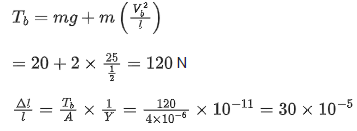Q.7. The diameter of an air bubble which was initially 2 mm, rises steadily through a solution of density 1750 kg m−3 at the rate of 0.35cms−1. The coefficient of viscosity of the solution is _________ poise (in nearest integer). (the density of air is negligible).    (JEE Main 2022)

Ans. 11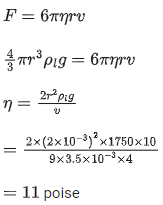Q.8. A spherical soap bubble of radius 3 cm is formed inside another spherical soap bubble of radius 6 cm. If the internal pressure of the smaller bubble of radius 3 cm in the above system is equal to the internal pressure of the another single soap bubble of radius r cm. The value of r is ___________.    (JEE Main 2022)

Ans. 2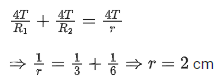Q.9. In an experiment to determine the Young's modulus, steel wires of five different lengths (1,2,3,4, and 5 m) but of same cross section (2 mm2) were taken and curves between extension and load were obtained. The slope (extension/load) of the curves were plotted with the wire length and the following graph is obtained. If the Young's modulus of given steel wires is x×1011Nm−2, then the value of x is __________.    (JEE Main 2022)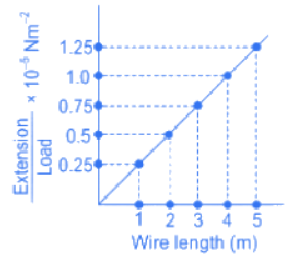Ans. 2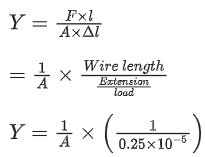Y = 1011 x 2
⇒ x = 2

Q.10. A square aluminum (shear modulus is 25 × 109Nm−2) slab of side 60 cm and thickness 15 cm is subjected to a shearing force (on its narrow face) of 18.0 × 104 N. The lower edge is riveted to the floor. The displacement of the upper edge is ____________ μm.    (JEE Main 2022)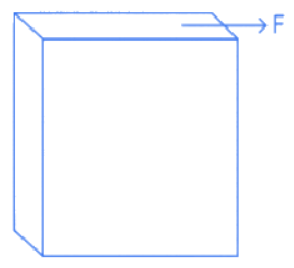Ans. 48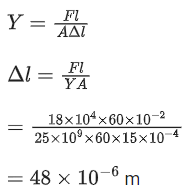Q.11. A uniform heavy rod of mass 20 kg, cross sectional area 0.4 m2 and length 20 m is hanging from a fixed support. Neglecting the lateral contraction, the elongation in the rod due to its own weight is x × 10−9 m. The value of x is _______________.
(Given, young modulus Y = 2 × 1011 Nm−2 and g = 10 ms−2)    (JEE Main 2022)

Ans. 25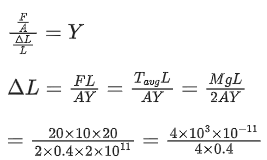Q.12. In an experiment to determine the Young's modulus of wire of a length exactly 1 m, the extension in the length of the wire is measured as 0.4 mm with an uncertainty of ± 0.02 mm when a load of 1 kg is applied. The diameter of the wire is measured as 0.4 mm with an uncertainty of ± 0.01 mm. The error in the measurement of Young's modulus (ΔY) is found to be x × 1010Nm−2. The value of x is _________________. (take g = 10 ms−2)    (JEE Main 2022)

Ans. 2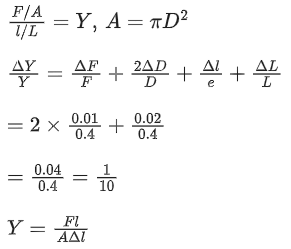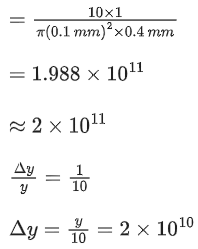Q.13. A unit scale is to be prepared whose length does not change with temperature and remains 20 cm, using a bimetallic strip made of brass and iron each of different length. The length of both components would change in such a way that difference between their lengths remains constant. If length of brass is 40 cm and length of iron will be __________ cm. iron (αiron = 1.2 × 10−5 K−1 and brass αbrass  = 1.8 × 10−5 K−1).    (JEE Main 2022)

Ans. 60
ΔL1 = α1L1ΔT
ΔL2 = α2L2ΔT
α1L1 = α2L2
1.2 × 10−5 × L1 = 1.8 × 10−5 × L2
L1 = 1.8/1.2 × 40 = 60 cm

Q.14. A wire of length L and radius r is clamped rigidly at one end. When the other end of the wire is pulled by a force F, its length increases by 5 cm. Another wire of the same material of length 4L and radius 4r is pulled by a force 4F under same conditions. The increase in length of this wire is __________________ cm.    (JEE Main 2022)

Ans. 5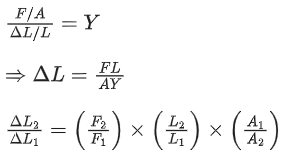= 4 × 4 × 1/16 = 1
ΔL2 = ΔL1 = 5 cm.

Q.15. The excess pressure inside a liquid drop is 500 Nm−2. If the radius of the drop is 2 mm, the surface tension of liquid is x × 10−3 Nm−1. The value of x is _____________.    (JEE Main 2022)

Ans. 500
P = P0 + 2T/R
⇒ P − P0 = 2T/R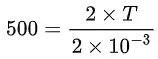T = 500 × 10−3
So, x = 500

Q.16. The velocity of upper layer of water in a river is 36 kmh−1. Shearing stress between horizontal layers of water is 10−3 Nm−2. Depth of the river is __________ m. (Co-efficient of viscosity of water is 10−2 Pa.s)    (JEE Main 2022)

Ans. 100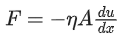⇒ 10−3 = 10−2 × 10/h
⇒ h = 10−1/10−3 m = 100 m
⇒ (100)

Q.17. An ideal fluid of density 800 kgm−3, flows smoothly through a bent pipe (as shown in figure) that tapers in cross-sectional area from a to α/2. The pressure difference between the wide and narrow sections of pipe is 4100 Pa. At wider section, the velocity of fluid is √x/6 ms−1 for x = ___________. (Given g = 10 ms−2)    (JEE Main 2022)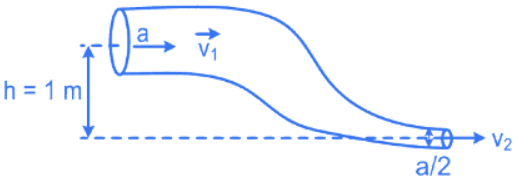Ans. 363
From Bernoulli's equation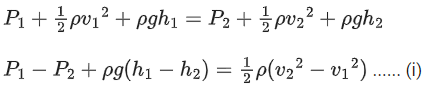Also, from equation of continuity
A1v1 = A2v2
Av1 = A/2v2
v2 = 2v1 ...... (ii)
put equation (ii) in (i),
4100 × 800 × 10 × 1 = 1/2 × 800 × (4v1− v12)
4100 + 8000 = 400 × 3v12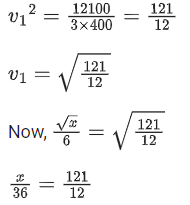x = 121 × 3 = 363
∴ x = 363

Q.18.  The elastic behaviour of material for linear stress and linear strain, is shown in the figure. The energy density for a linear strain of 5 × 10−4 is __________ kJ/m3. Assume that material is elastic upto the linear strain of 5 × 10−4.    (JEE Main 2022)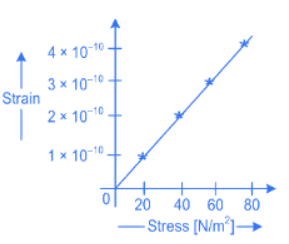Ans. 25
slope of strain − stress curve given by = 10−10/20
for strain of 5 × 10−4 stress is given by
5 × 10−4 = 10−10/20 × stress
stress = 108 N/m2
Energy density  =1/2 × strain × stress
= 1/2 × 5 × 10−4 × 108
= 25000 J/m3
= 25 kJ/m3

Q.19. The area of cross-section of a large tank is 0.5 m2. It has a narrow opening near the bottom having area of cross-section 1 cm2. A load of 25 kg is applied on the water at the top in the tank. Neglecting the speed of water in the tank, the velocity of the water, coming out of the opening at the time when the height of water level in the tank is 40 cm above the bottom, will be ___________ cms−1. [Take g = 10 ms−2]    (JEE Main 2022)

Ans. 300
By Bernoulli's theorem: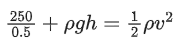⇒ v = 3 m/s
⇒ v = 300 cm/s

Q.20. A liquid of density 750 kgm−3 flows smoothly through a horizontal pipe that tapers in cross-sectional area from A1 = 1.2 × 10−2 m2 to A2 = A1/2. The pressure difference between the wide and narrow sections of the pipe is 4500 Pa. The rate of flow of liquid is ___________ × 10−3 m3s−1.    (JEE Main 2022)

Ans. 24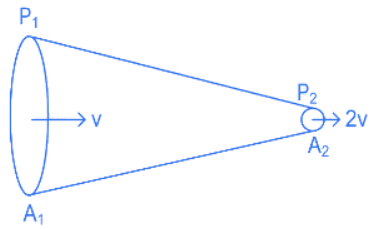Using Bernoulli's equation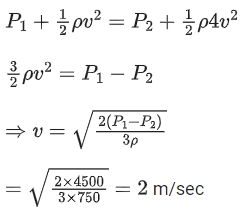So Q = A1v = 24 × 10−3 m3/sec

Q.21. A small spherical ball of radius 0.1 mm and density 104 kg m−3 falls freely under gravity through a distance h before entering a tank of water. If, after entering the water the velocity of ball does not change and it continue to fall with same constant velocity inside water, then the value of h will be ___________ m.
(Given g = 10 ms−2, viscosity of water = 1.0 × 10−5 N-sm−2).    (JEE Main 2022)

Ans. 20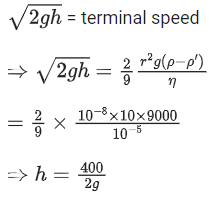⇒ h = 20 m

Q.22. If the length of a wire is made double and radius is halved of its respective values. Then, the Young's modulus of the material of the wire will :    (JEE Main 2022)
(a) remain same
(b) become 8 times its initial value
(c) become 1/4 of its initial value
(d) become 4 times its initial value

Ans. a
Young's modulus of matter depends on material of wire and is independent of the dimensions of the wire. As the material remains same so Young's modulus also remain same.

Q.23. Given below are two statements : One is labelled as Assertion (A) and the other is labelled as Reason (R).
Assertion (A): Clothes containing oil or grease stains cannot be cleaned by water wash.
Reason (R): Because the angle of contact between the oil/grease and water is obtuse.
In the light of the above statements, choose the correct answer from the option given below.    (JEE Main 2022)
(a) Both (A) and (R) are true and (R) is the correct explanation of (A)
(b) Both (A) and (R) are true but (R) is not the correct explanation of (A)
(c) (A) is true but (R) is false
(d) (A) is false but (R) is true

Ans. a
Due to obtuse angle of contact the water doesn't wet the oiled surface properly and cannot wash it also.
⇒ Assertion is correct and Reason given is a correct explanation.

Q.24. Given below are two statements : One is labelled as Assertion (A) and other is labelled as Reason (R).
Assertion (A) : Time period of oscillation of a liquid drop depends on surface tension (S), if density of the liquid is ρ and radius of the drop is r, then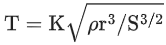is dimensionally correct, where K is dimensionless.Reason (R) : Using dimensional analysis we get R.H.S. having different dimension than that of time period.In the light of above statements, choose the correct answer from the options given below.    (JEE Main 2022)
(a) Both (A) and (R) are true and (R) is the correct explanation of (A)
(b) Both (A) and (R) are true but (R) is not the correct explanation of (A)
(c) (A) is true but (R) is false
(d) (A) is false but (R) is true

Ans. d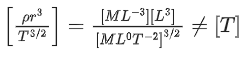As the equation for first statement is wrong dimensionally.
⇒ A is false and R is true.

Q.25. Consider a cylindrical tank of radius 1 m is filled with water. The top surface of water is at 15 m from the bottom of the cylinder. There is a hole on the wall of cylinder at a height of 5 m from the bottom. A force of 5 × 105 N is applied an the top surface of water using a piston. The speed of ifflux from the hole will be : (given atmospheric pressure PA = 1.01 × 105 Pa, density of water ρW = 1000 kg/m3 and gravitational acceleration g = 10 m/s2)    (JEE Main 2022)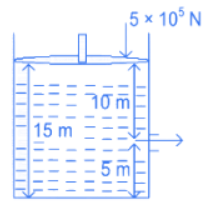(a) 11.6 m/s
(b) 10.8 m/s
(c) 17.8 m/s
(d) 14.4 m/s

Ans. c
By Bernoulli's theorem,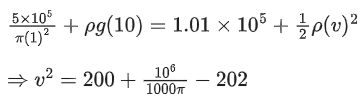⇒ v ≃ 17.8 m/s

Q.26. A pressure-pump has a horizontal tube of cross sectional area 10 cm2 for the outflow of water at a speed of 20 m/s. The force exerted on the vertical wall just in front of the tube which stops water horizontally flowing out of the tube, is :    (JEE Main 2022)
[given: density of water = 1000 kg/m3]
(a) 300 N
(b) 500 N
(c) 250 N
(d) 400 N

Ans. d
Fw = ρAv2
= 103 × 10 × 10−4 × 20 × 20
= 400N

Q.27. The force required to stretch a wire of cross-section 1 cm2 to double its length will be : (Given Yong's modulus of the wire = 2 × 1011 N/m2)    (JEE Main 2022)
(a) 1 × 107 N
(b) 1.5 × 107 N
(c) 2 × 107 N
(d) 2.5 × 107 N

Ans. c
A = 1 cm2
Y = Fl/AΔl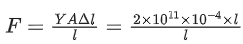= 2 × 107 N

Q.28. A balloon has mass of 10 g in air. The air escapes from the balloon at a uniform rate with velocity 4.5 cm/s. If the balloon shrinks in 5 s completely. Then, the average force acting on that balloon will be (in dyne).    (JEE Main 2022)

(a) 3
(b) 9
(c) 12
(d) 18

Ans. b
Favg = μ × vrel
=10/5 × 4.5 = 9

Q.29. A steel wire of length 3.2 m(Ys = 2.0 × 1011 Nm−2) and a copper wire of length 4.4 m(Yc = 1.1 × 1011Nm−2), both of radius 1.4 mm are connected end to end. When stretched by a load, the net elongation is found to be 1.4 mm. The load applied, in Newton, will be: ( Given π = 22/7)    (JEE Main 2022)
(a) 360
(b) 180
(c) 1080
(d) 154

Ans. d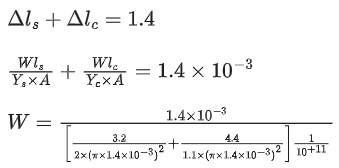W ≃ 154 N

Q.30. Two cylindrical vessels of equal cross-sectional area 16 cm2 contain water upto heights 100 cm and 150 cm respectively. The vessels are interconnected so that the water levels in them become equal. The work done by the force of gravity during the process, is [Take, density of water = 103 kg/m3 and g = 10 ms−2] :    (JEE Main 2022)
(a) 0.25 J
(b) 1 J
(c) 8 J
(d) 12 J

Ans. b
A = 16 × 10−4 m2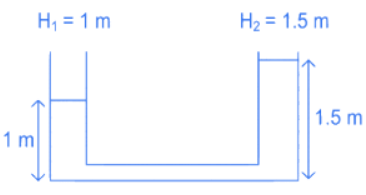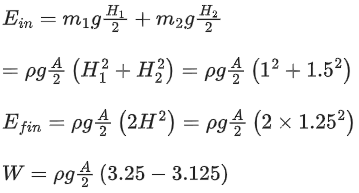= 1 J

Q.31. A water drop of radius 1 cm is broken into 729 equal droplets. If surface tension of water is 75 dyne/ cm, then the gain in surface energy upto first decimal place will be :
(Given π = 3.14 )    (JEE Main 2022)
(a) 8.5 × 10−4 J
(b) 8.2 × 10−4 J
(c) 7.5 × 10−4 J
(d) 5.3 × 10−4 J

Ans. c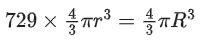⇒ R = 9r ........ (1)
ΔU = S × ΔA ..... (2)
⇒ ΔU = S × {−4πR2 + 729 × 4πr2}
= S × 4π{729r− 81r2}
= 7.5 × 10−4J

Q.32. A drop of liquid of density ρ is floating half immersed in a liquid of density σ and surface tension 7.5 × 10−4 Ncm−1. The radius of drop in cm will be :    (JEE Main 2022)
(g = 10 ms−2)
(a)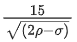(b)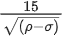(c)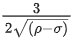(d)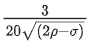Ans. a
Balancing the forces on drop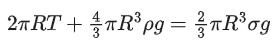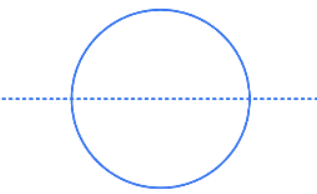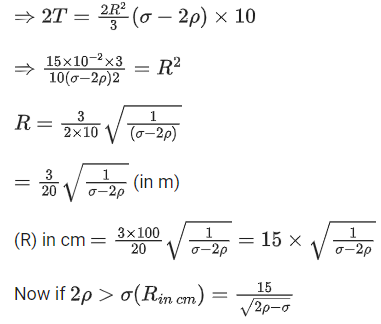Q.33. An air bubble of negligible weight having radius r rises steadily through a solution of density σ at speed v. The coefficient of viscosity of the solution is given by :    (JEE Main 2022)
(a) η = 4rσg/9v
(b) η = 2r2σg/9v
(c) η = 2πr2σg/9v
(d) η = 2r2σg/3πv

Ans. b
Air bubble moves with constant speed v. So net force = 0.
∴ Buoyant Force = Viscous force
⇒ Fb = Fv
⇒ σ × 4/3πr3g = 6πnrv
⇒ n = 2σr2g/9v

Q.34. The bulk modulus of a liquid is 3 × 1010 Nm−2. The pressure required to reduce the volume of liquid by 2% is :    (JEE Main 2022)
(a) 3 × 108 Nm−2
(b) 9 × 108 Nm−2
(c) 6 × 108 Nm−2
(d) 12 × 108 Nm−2

Ans. c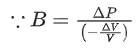⇒ ΔP = 3 × 1010 × (0.02)
= 6 × 108 N/m2

Q.35. The terminal velocity (vt) of the spherical rain drop depends on the radius (r) of the spherical rain drop as :    (JEE Main 2022)
(a) r1/2
(b) r
(c) r2
(d) r3

Ans. c
6πηvtr = 4/3πr3(ρ−σ)g
⇒ v= Cr2 where C is a constant
or vt ∝ r2

Q.36. A solid metallic cube having total surface area 24 m2 is uniformly heated. If its temperature is increased by 10C, calculate the increase in volume of the cube. (Given α = 5.0 × 10−4 C−1).    (JEE Main 2022)
(a) 2.4 × 106 cm3
(b) 1.2 × 105 cm3
(c) 6.0 × 104 cm3
(d) 4.8 × 105 cm3

Ans. b
6 × l2 = 24
⇒ l = 2 m
∴ ΔV/V = 3 × Δl/l
⇒ ΔV = 3 × (αΔT) × V
= 3 × 5 × 10−4 × 10 × (8)
= 120 × 10−3 m3
= 120 × 10−3 × 106 cm3
= 1.2 × 105 cm3

Q.37. If p is the density and η is coefficient of viscosity of fluid which flows with a speed v in the pipe of diameter d, the correct formula for Reynolds number Re is :    (JEE Main 2022)
(a) Re = ηd/ρv
(b) Re = ρv/ηd
(c) Re = ρvd/η
(d) Re = η/ρvd

Ans. c
Re = ρvd/η
Direct formula based.

Q.38. The velocity of a small ball of mass 'm' and density d1, when dropped in a container filled with glycerin, becomes constant after some time. If the density of glycerin is d2, then the viscous force acting on the ball, will be :    (JEE Main 2022)
(a)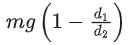(b)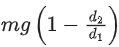(c)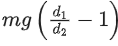(d)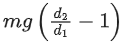Ans. b
Viscous force acting on the ball will be equal and opposite to net of weight and buoyant force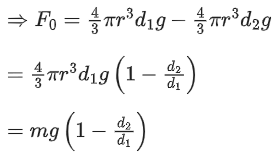Q.39. A water drop of diameter 2 cm is broken into 64 equal droplets. The surface tension of water is 0.075 N/m. In this process the gain in surface energy will be :    (JEE Main 2022)
(a) 2.8 × 10−4 J
(b) 1.5 × 10−3 J
(c) 1.9 × 10−4 J
(d) 9.4 × 10−5 J

Ans. a
r′ = r/4
⇒ ΔE = T(ΔS)
= T × 4π(nr′2−r2), n = 64
= T × 4π × (4 − 1)r2
⇒ ΔE = 0.075 × 4 × 3.142(3) × 10−4 J
= 2.8 × 10−4 J

Q.40. Given below are two statements : One is labelled as Assertion A and the other is labelled as Reason R.
Assertion A : Product of Pressure (P) and time (t) has the same dimension as that of coefficient of viscosity.
Reason R : Coefficient of viscosity = Force/Velocitygradient
Choose the correct answer from the options given below :    (JEE Main 2022)
(a) Both A and R are true, and R is the correct explanation of A.
(b) Both A and R are true but R is NOT the correct explanation of A.
(c) A is true but R is false.
(d) A is false but R is true.

Ans. c
[Pressure][Time] = [Force/Area] [distance/Area]
[Coefficient of viscosity] = [Force/Area] [distance/Area]
Statement 'A' is true
But Statement 'R' is false are coefficient of viscosity

The document JEE Main Previous year questions (2021-22): Properties of Matter - 1 - Notes | Study Physics 35 Years JEE Main & Advanced Past year Papers - JEE is a part of the JEE Course Physics 35 Years JEE Main & Advanced Past year Papers.
All you need of JEE at this link: JEE

## Physics 35 Years JEE Main & Advanced Past year Papers

110 docs|49 tests
 Use Code STAYHOME200 and get INR 200 additional OFF

## Physics 35 Years JEE Main & Advanced Past year Papers

110 docs|49 tests

Track your progress, build streaks, highlight & save important lessons and more!

,

,

,

,

,

,

,

,

,

,

,

,

,

,

,

,

,

,

,

,

,

;